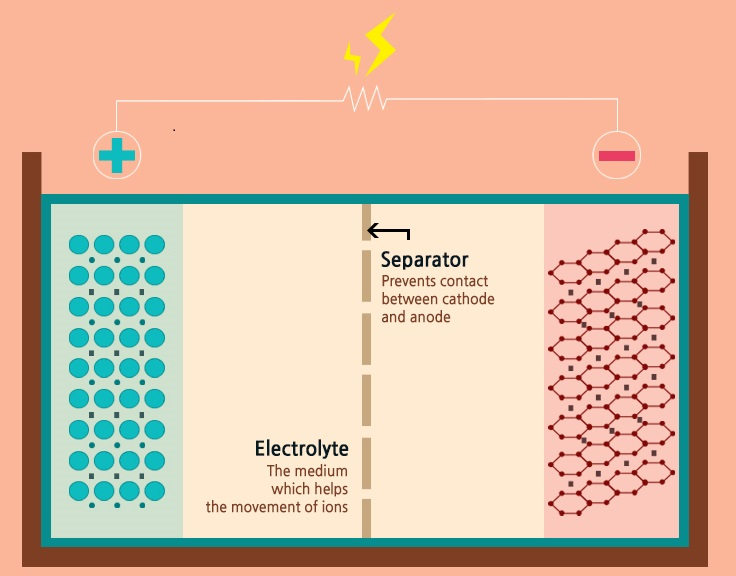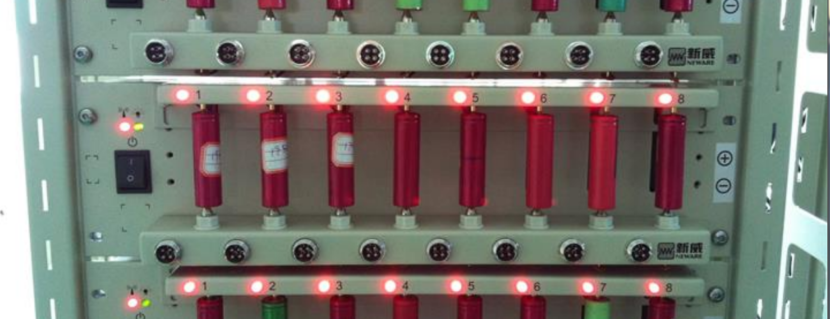# Master's Certification Program in Electric Vehicle Design & Analysis

8 month long Master's certification program in Electric Vehicle Design & Analysis

• Domain : EV, Instrumentation, BMS
• Pre-requisites : For Electrical, Electronics and Instrumentation Engineers### Program Outcomes

This program includes courses dealing with both EV as a system and EV as a system of systems.
Students will carry out a system-level simulation of an entire EV using MATLAB and Simulink in the first half of the Master Program. Afterward, they learn about the development of individual systems: Battery, Motor, and Power converter.
This Master's Program enhances system design, circuit design, PCB design, and motor design skills which will educate the students in most of the EV design aspects.

After completing this program, students will be eligible to apply for the post of a simulation engineer, system engineer, hardware and software engineer, and similar vacancies at both Automotive & related components supplier companies. They can also choose to go for startup jobs in the relevant fields. The students will also get opportunities in research centers at Universities or similar consultancy organizations.

These are some of the organizations recruiting students upon their completion of this Master's Program - Mahindra, Tata Motors, Ford, Bajaj, TVS, Maruti, Force, Renault, Valeo, Hella, Varroc, Motherson, Greaves cotton, Uno Minda, Ather energy, Ola electric, Yulu, Magneti Marelli, Magna, Delphi, Denso, Continental.### Program Timeline

The entire program is split into two phases. During the first phase, you will be taking the mandatory courses. These courses are fundamental for anyone who wishes to pursue their career in the Electric Vehicle Domain.

Phase 1

1. Simulink for Mechanical & Electrical Engineers
2. Machine learning for Electrical Engineers using Python
3. Introduction to Hybrid Electric Vehicle using MATLAB and Simulink
4. Introduction to Control of Electric Vehicle
5. Introduction to Battery Technology for Electric Vehicle
6. Fuel cell and Ultra capacitor for EV using MATLAB & Simulink

Phase 2

2. Introduction to Automotive Embedded Systems & AUTOSAR
3. Analysis & Simulation of Power Converters for EV using MATLAB/Simulink and LTSpice
4. Digital control of power converters with C2000 using Altair Embed
5. PCB design for beginners using Altium designer
6. Basics of Electromagnetics and design of BLDC motor using ANSYS

In addition to the above courses, new electives might be offered to the student. If you have already registered for the program then there is no additional charge for the new electives.

Job Assistance

Job assistance is automatically enabled for students who complete a minimum of 3 courses. Completion is defined by video watch time(90%), challenge completion (80%), and maintaining a good technical profile in SKILL-LYNC

# GET COURSE COUNSELLING TODAY

Speak to our technical specialists to understand what is included in this program and how you can benefit from it.### Simulink for Electrical & Mechanical Engineers

#### 1 Introduction to modelling of complex systems

• Importance of mathematical modelling
• Block diagram approach & model-based design for engineering systems
• Creating a simple model
• Obtaining results
• Introduction to various Simulink toolboxes

#### 2Simulation configurations & Simscape

• How does a model run in Simulink?
• Types of solvers
• Model configuration
• Continuous & discrete-time systems
• Timestep
• Solving ODE
• Introduction to physical modelling using simscape

#### 3Simulink with script and workspace

• Using a script file with Simulink models
• Creating a subsystem
• Variant subsystem
• Projects
• Template
• lookup tables
• Running simulation in steps

#### 4Stateflow for control logic

• Control logic for engineering applications
• Using a finite state machine
• Making logic diagrams### Machine learning for Electrical Engineers using Python

#### 1Basics of Probability and Statistics

In this module, we will introduce the ideas of Statistics, machine learning and artificial intelligence.

Basics of Probability

Basics of Statistics

What is ML & AI

#### 2Basics of ML & AL

Introduction to normal distribution & standard normal distribution

Artificial Intelligence

#### 3Supervised Learning - Prediction

Introduction to supervised learning

What is linear regression

One hot encoding

#### 4Supervised Learning - Classification

Introduction to classification problems

What is logistic regression

#### 5Supervised Learning - Classification

In this module, we will introduce some more classification algorithms

Decision tree

Entropy

Information gain

#### 6Random forest & Model Evaluation

In this section, we will assess the algorithms

Random Forest

Bootstrapping and majority rule

Evaluation of classifiers

#### 7Supervised Learning - Classification

In this module, we will introduce SVM

Support Vector Machines

Mathematical intuition behind SVM

How SVM is different from other classifiers

#### 8Supervised Learning - Classification

In this section, we will introduce knn

K-Nearest Neighbour

Lazy Algorithm

Single layer Neural Network

#### 9Unsupervised Learning - Kmeans

In this section, we will introduce clustering

What is clustering

Why clustering is important

Kmeans and elbow curve

#### 10Unsupervised Learning - Hierarchical

In this section, we will introduce another type of clustering

Hierarchical Clustering

Dendrogram

Evaluation of clustering algorithms

#### 11Unsupervised Learning - PCA

In this module, we will introduce some feature selection techniques

Feature Selection

Principal Component Analysis

Mathematical intuition behind PCA

#### 12Supervised Learning - Classification

In this section, we will introduce neural network

Artificial Neural Network

Deep learning

Different activation functions

Understanding back propagation### Introduction to Hybrid Electric Vehicle using MATLAB and Simulink

#### 1Traction Power Equations, Driving Cycle & Importance of Modelling

The fundamentals of vehicle design involve the basic principles of physics. The vehicle motion can be completely determined by analysing the forces acting on it in the direction of motion.The response of the vehicle for different drive cycle patterns along with wide-open throttle calculations are discussed in this session.

• Vehicle power requirement calculations: Rolling resistance, air drag, hill climbing and acceleration forces
• Driving patterns and vehicle testing standards, important terms and definitions related to driving cycle, Energy expenditure calculation

#### 2Hybrid Electric Vehicle Architectures

The General EV and HEV configuration is proposed unequivocally. The generic concept of a hybrid drivetrain and possible energy flow route helps to understand the challenges of battery charging and power consumption. The advanced simulator tool (ADVISOR tool) gives an idea of a detailed profile for vehicle modeling and reasoning.

• Different configurations of power train components: Battery Electric Vehicle, Hybrid Electric Vehicle: Series, Parallel and Series-Parallel
• MATLAB Simulation: ADVISOR and Powertrain block set
• Various modelling options, Control strategies

#### 3Electric Motors & Power Converters for Propulsion

In a motor vehicle, the powertrain or powerplant comprises the main components that generate power and deliver that power to the road. In hybrid powertrains, the torque generated by the combustion engine and the electric motor has to be brought together and distributed to the wheels. The control of this power flow is discussed along with the working of electric motors.

Electrical engineering terminologies, Fundamentals of DC and AC motors: working principle, characteristics, and control.

• Understanding power electronics, basic DC converter circuit operation, power devices, switching losses
• Power electronic control of PMDC, PMBLDC and induction motor
• Inverter operation, Sine PWM, Variable frequency control
• Review of induction motor controller, connections and features, limitations of variable frequency control, introduction to vector control

#### 4Braking of Electrical Motors & Motor Efficiency Plots

Moving vehicles have a lot of kinetic energy, and when brakes are applied to slow a vehicle, all of that kinetic energy has to go somewhere. Regenerative braking uses an electric vehicle’s motor as a generator to convert much of the kinetic energy lost when decelerating back into stored energy in the vehicle’s battery. To evaluate regenerative braking, we really need to look at two different parameters, efficiency and effectiveness.

• Braking requirements of vehicle, Methods of braking of DC & Induction motors: regenerative braking and dynamic braking
• Coordinating electrical and mechanical brakes, braking control strategies### Introduction to Control of Electric Vehicle

#### 1Introduction to control system

Fundamental control components are discussed in this section. Students can learn the basics of control system Engineering from this section right from types of the control system to various components of control theories.

• Fundamentals of control engineering
• Important terms
• Feedback system
• Transfer functions
• Poles zeros
• State-space
• Laplace transform

#### 2Stability analysis

This section dealt with the stability analysis of control systems. It is a prerequisite for any of the systems to be stable to give satisfactory behaviour. Students can learn about stability theories and how to implement the same using the MATLAB command.

• Stability
• Bode plot and MATLAB tools for control system engineering

#### 3Control theory applied to power converters and motors

A PID controller is considered to be very important in the industrial standpoint. This section helps to learn the significance of the PID controller and how to auto-tune the gain parameters of the PID controller in MATLAB simulation environment.

• PID control actions and simulations for step response

#### 4Controller design for DC motor

In an Electric Vehicle, the motor plays a crucial part to propel the vehicle. The motor characteristic needs to be known to control it efficiently. This section helps to learn about the transfer function of DC motors and control of DC-DC power converter.

• DC motor transfer function
• Setpoint tracking and disturbance rejection

#### 5Introduction to Digital Control

This section briefs the difference between analogue and digital systems. The significance of discrete control systems and the domain that is to be defined for the discrete control system. Student can get an insight on the advantage of using Discrete control system over the Analog control system

• Limitations of analog control
• Discrete control system
• Z-domain analysis

#### 6Using Microcontroller for Motor Control

Students can learn the fundamentals of microprocessors and microcontrollers. This section gives knowledge about the architecture of Microcontroller and how it can be programmed and interfaced.
• Digital power control
• Microcontroller fundamentals
• Programming and interface

#### 7Vehicle Dynamics Control

Vehicle Dynamics Control System Algorithm is significant in the Connected Cars System & using such algorithms with data interaction between sensor & actuators help the engineers to maintain the safety & security of the vehicle. .The importance of a Master Controller and how it is interfaced with other controllers is also discussed in this topic.
• Intelligent and connected vehicle system
• Master controller and its interfacing with the local controller### Introduction to Battery Technology for Electric Vehicle

#### 1Energy and Electrochemistry

• Sources of energy for propulsion & their comparison: Net Calorific Value, Conversion efficiency

• History and background of battery technology

• Electrochemistry fundamentals & terminologies

• Lithium ion battery and different chemistries#### 2Important Terms and Characteristics

• Portable power applications and electrical load requirements

• Factors affecting the choice of EV battery systems

• Commercially available lithium ion cells

• Electrical characteristics of battery: Capacity, C-rate, impedance, DOD, SOC, SOH, Life cycles, Mechanical characteristics, Form factor, Safety

#### 3Mathematical Modelling